Function Repository Resource:

# FromInversionVector

Construct the permutation list corresponding to the given inversion vector

Contributed by: Wolfram Staff
 ResourceFunction["FromInversionVector"][vect] constructs the permutation list corresponding to the inversion vector vect.

## Details and Options

The inversion vector of a permutation of length n lists the number of times k is preceded by an entry greater than k, where k runs from 1 to n.

## Examples

### Basic Examples (2)

Here is the inversion vector of a permutation:

 In:=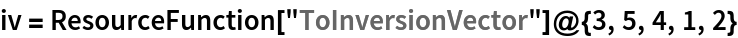Out=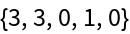This gives back the permutation:

 In:=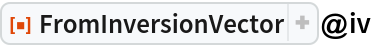Out=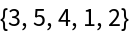### Scope (3)

Here are all six permutation of {1,2,3}:

 In:=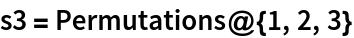Out=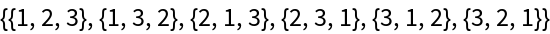Here are the corresponding inversion vectors:

 In:=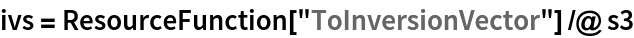Out=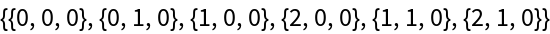The round trip works:

 In:=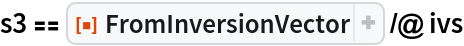Out=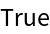George Beck

## Version History

• 2.0.0 – 08 July 2019
• 1.0.0 – 31 May 2019

## Author Notes

The Combinatorica` function ToInversionVector did not include the final zero.